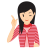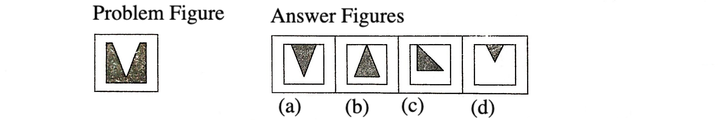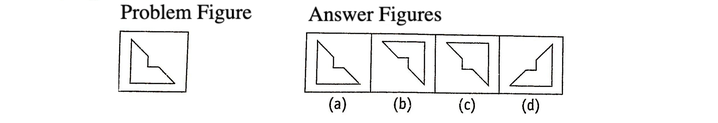Square Completion

" Square Completion is based on formation of a square by joining the piece given in the problem figure with an another piece given in one of the options " .

TIPS on cracking Non Verbal Reasoning Questions on Square CompletionFew Important things to Remember

Time is a very important thing in every exams. You should complete your exam within the time frame. But in a competitive exam, question are for testing your calculation ability within a given time frame. They tests, how quickly a student can solve a question paper. This is the reason so many students couldn’t finish their paper within given time. But if you need to solve your government or bank or any other question paper quickly then you should use tips of reasoning and Square Completion (Non Verbal Reasoning) which will help you to solve government, bank and other exam papers quickly.

Tip# : In the questions based on square completion, a problem figure which represents an incomplete square is given, followed by four answer figures and only one out of these four answer figures is appropriate to make a complete square with the piece given in the problem figure. A candidate is required to find out the correct answer figure. This section can be better understood through paper and cardboard cut pieces.

Following examples will provide you a clear idea about the pattern of questions asked in various exams

Directions (Example Nos. 1-2) In the following questions, a problem figure is given followed by four answer figures. You have to choose that answer figure which on joining will make a complete square with the problem figure.

Example 1: Choose the correct answer figure which will make a complete square on joining with the problem figure.Solution:  (a) By careful examination of all the answer figures, it is clear that option (a) is the correct answer figure which will fill the blank portion and make a complete square with the problem figure. This is shown as belowExample 2: Choose the correct answer figure which will make a complete square on joining with the problem figure.Solution:  (c) By careful examination of all the answer figures, it is clear that option (c) is the correct answer figure which will make a complete square with the problem figure. This is shown as belowLearnFrenzy provides you lots of fully solved Square of Completion Questions and Answers with explanation.